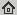# Rotational Invariance Based on Fourier Analysis in Polar and Spherical Coordinates

IEEE Transactions on Pattern Analysis and Machine Intelligence, 31(9): 1715--1722, 2009
Abstract: In this paper, polar and spherical Fourier analysis are defined as the decomposition of a function in terms of eigenfunctions of the Laplacian with the eigenfunctions being separable in the corresponding coordinates. The proposed transforms provide effective decompositions of an image into basic patterns with simple radial and angular structures. The theory is compactly presented with an emphasis on the analogy to the normal Fourier transform. The relation between the polar or spherical Fourier transform and the normal Fourier transform is explored. As examples of applications, rotation-invariant descriptors based on polar and spherical Fourier coefficients are tested on pattern classification problems.

## BibTex reference

```@Article{WRB09,
author       = "Q.Wang and O.Ronneberger and H.Burkhardt",
title        = "Rotational Invariance Based on Fourier Analysis in Polar and Spherical Coordinates",
journal      = "IEEE Transactions on Pattern Analysis and Machine Intelligence",
number       = "9",
volume       = "31",
pages        = "1715--1722",
year         = "2009",
keywords     = "Invariants, Fourier analysis, radial transform, multidimensional",
url          = "http://lmb.informatik.uni-freiburg.de/Publications/2009/WRB09"
}
```